# Math in Focus Grade 6 Chapter 5 Lesson 5.1 Answer Key Rates and Unit Rates

Go through the Math in Focus Grade 6 Workbook Answer Key Chapter 5 Lesson 5.1 Rates and Unit Rates to finish your assignments.

## Math in Focus Grade 6 Course 1 A Chapter 5 Lesson 5.1 Answer Key Rates and Unit Rates

### Math in Focus Grade 6 Chapter 5 Lesson 5.1 Guided Practice Answer Key

State whether each statement is expressed as a unit rate.

Question 1.
A monkey plucked 4 coconuts per minute.
yes, it can be expressed in unit ratio.
Explanation:
Statement is expressed as a unit rate.
per minute is unit

Question 2.
Mary paid $2 for a bottle of orange juice. Answer: Yes, it can be expressed in unit ratio. Explanation:$2 for a bottle of orange juice.
$is unit per bottle juice. Question 3. A basketball team scored 294 points in 6 games. Answer: yes, it can be expressed in unit ratio. Explanation: points are the units for games Question 4. Douglas reads 3 books in a week. Answer: Yes, it can be expressed in unit ratio. Explanation: Week is unit for reading a book. Solve. Question 5. A photocopy machine can print 405 copies in 9 minutes. What is the rate at which the machine prints the copies?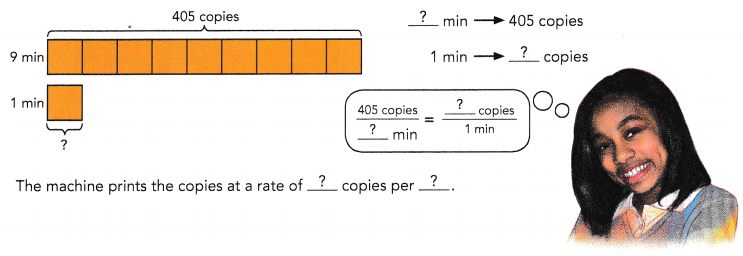Answer: 45 copies per min Explanation: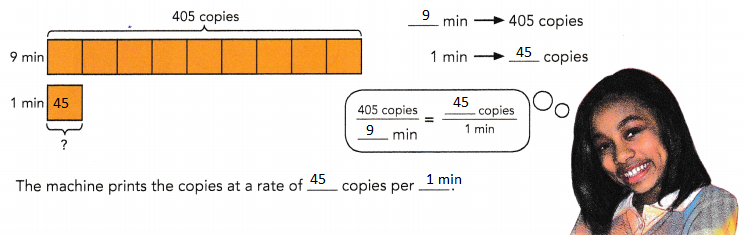Question 6. A sports center buys 1.25 acres of land for a new swimming complex. What is the cost per acre if the sports center pays$100,000 for the land?
Cost per acre = Total cost ÷ Total number of acres
= $100,000 ÷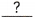=$$$\frac{100,000}{?}$$
= $$$\frac{?}{?}$$ =$The unit cost of the piece of land is $per acre. Answer:$ 80,000 per acre.
Explanation:
Cost per acre = Total cost ÷ Total number of acres
= $100,000 ÷ 1.25 =$$$\frac{100,000}{1.25}$$
= $$$\frac{1,000,000}{125}$$ =$ 80,000
The unit cost of the piece of land is $80000 per acre. Question 7. A few years ago, when the fuel tank in Sally’s car was completely empty, she paid$63 to fill the tank with 22.5 gallons of gasoline. What was the cost per gallon?
Cost per gallon = Cost of gasoline ÷ Volume of gasoline filled
=$÷=$The unit cost of the gasoline was $per gallon. Answer:$2.8
Explanation:
Cost per gallon = Cost of gasoline ÷ Volume of gasoline filled
=$63 ÷ 22.5 =$ 2.8
The unit cost of the gasoline was $2.8 per gallon. Question 8. The table shows the costs of some food items Billy bought from a supermarket.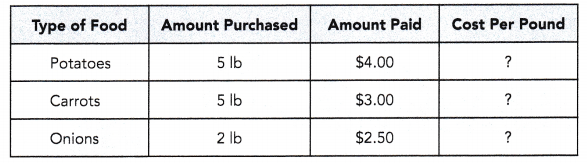Answer: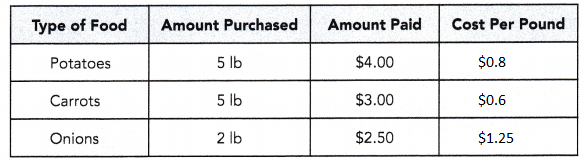Which type of food costs the most per pound? Cost of potatoes per pound = Cost of potatoes ÷ Weight of potatoes =$÷= $The unit cost of the potatoes is$per pound.
Cost of carrots per pound = Cost of carrots ÷ Weight of carrots
= $÷=$The unit cost of the carrots is $per pound. Cost of onions per pound = Cost of onions ÷ Weight of onions =$÷= $The unit cost of the onions is$per pound.
Comparing the unit costs of the food items, the unit cost of theis the greatest.
Unit cost of < Unit cost of< Unit cost of$<$< $So, thecost the most per pound. Answer:$1.25 cost the most per pound.
Explanation:
Cost of potatoes per pound = Cost of potatoes ÷ Weight of potatoes
= $4 ÷ 5 =$ 0.8
The unit cost of the potatoes is $0.8 per pound. Cost of carrots per pound = Cost of carrots ÷ Weight of carrots =$3 ÷ 5
= $0.6 The unit cost of the carrots is$ 0.6 per pound.
Cost of onions per pound = Cost of onions ÷ Weight of onions
= $2.5 ÷ 2 =$ 1.25
The unit cost of the onions is $1.25 per pound. Comparing the unit costs of the food items, the unit cost of the 1.25 is the greatest. Unit cost of Carrot < Unit cost of Potatoes < Unit cost of Onions$0.6 < $0.8 <$1.25
So, the $1.25 cost the most per pound. Question 9. A truck traveled a distance of 280 kilometers in 4 hours. Find the speed of the truck. Method 1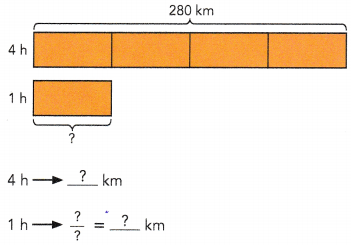The speed of the truck is 70kilometers per hour. Method 2 Speed of truck = $$\frac{\text { Distance }}{\text { Time }}$$ = $$\frac{?}{?}$$ =km/h The speed of the truck iskilometers per hour. Answer: 70 kilometers per hour. Explanation: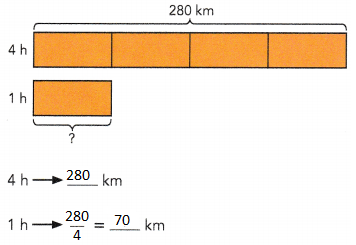Speed of truck = $$\frac{\text { Distance }}{\text { Time }}$$ = $$\frac{280}{4}$$ = 70 km/h The speed of the truck is 70 kilometers per hour. Question 10. A car travels $$\frac{1}{4}$$ kilometer in $$\frac{1}{2}$$ minute. Find the speed of the car in kilometers per minute. Answer: $$\frac{1}{2}$$ kilometers per minute. Explanation: Speed of car = $$\frac{\text { Distance }}{\text { Time }}$$ Speed of car = $$\frac{1}{4}$$ ÷ $$\frac{1}{2}$$ = $$\frac{1}{4}$$ X $$\frac{2}{1}$$ = $$\frac{1}{2}$$ kilometers per minute. ### Math in Focus Course 1A Practice 5.1 Answer Key Solve. Show your work. Question 1. A machine can print 300 T-shirts in 10 minutes. How many T-shirts can the machine print in 1 minute? Answer: 30 T-shirts Explanation: A machine can print 300 T-shirts in 10 minutes. Number of T-shirts can the machine print in 1 minute = $$\frac{300}{10}$$ = 30 T-shirts can the machine print in 1 minute Question 2. Alisa types 900 words in 20 minutes. What is her typing speed in words per minute? Answer: 45 words per minute Explanation: Alisa types 900 words in 20 minutes. Her typing speed in words per minute is, = $$\frac{900}{20}$$ = 45 words per minute Question 3. A 2-liter bottle is filled completely with water from a faucet in 10 seconds. How much water is filled into the bottle each second? Answer: 200 ml bottle each second Explanation: A 2-liter bottle is filled completely with water from a faucet in 10 seconds. water is filled into the bottle each second = $$\frac{2000}{10}$$ = 200 ml bottle each second Question 4. Bill is paid$200 for 5 days of work. How much is he paid per day?
$40 per day Explanation; Bill is paid$200 for 5 days of work.
Total amount he paid per day
= $$\frac{200}{5}$$
= $40 per day Question 5. Janice swims 450 meters in 5 minutes. Find her swimming speed in meters per minute. Answer: 90 meters per minute. Explanation: Janice swims 450 meters in 5 minutes. Her swimming speed in meters per minute. = $$\frac{450}{5}$$ = 90 meters per minute. Question 6. A garden snail moves $$\frac{1}{6}$$ foot in $$\frac{1}{3}$$ hour. Find the speed of the snail in feet per hour. Answer: $$\frac{1}{2}$$ feet per hour Explanation: A garden snail moves $$\frac{1}{6}$$ foot in $$\frac{1}{3}$$ hour. The speed of the snail in feet per hour. $$\frac{1}{6}$$ ÷ $$\frac{1}{3}$$ = $$\frac{1}{6}$$ X $$\frac{3}{1}$$ = $$\frac{1}{2}$$ feet per hour Question 7. Math Journal A plumber pays$3.60 for 60 centimeters of pipe. Explain how the plumber can use the unit cost of the pipe to find the cost of buying 100 meters of the same kind of pipe. Show the calculations the plumber needs to make.
The cost of 100 meters pipe = $600 Explanation: A plumber pays$3.60 for 60 centimeters of pipe.
1 cm pipe = 3.60 ÷ 60
= 0.6 cm
1m = 100 cm
S0, 0.6 cm = 600

Question 8.
Rovan can make 48 tarts per hour. Copy and complete the table.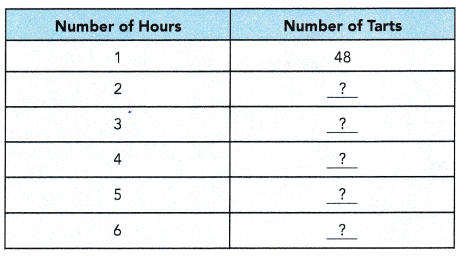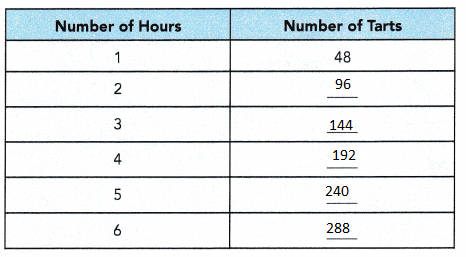a) At this rate, how many tarts can Rovan make in 6 hours?
288 Tarts
Explanation:
From the above given information,
Number of tarts Rovan can make in 6 hours
6 x 48 = 288 tarts

b) At this rate, how long will she take to make 120 tarts?
2.5 hours
Explanation:
From the above given information,
Number of hours she take to make tarts = 120 ÷ 48
= 2.5 hours

Question 9.
A sprinkler system is designed to water $$\frac{5}{8}$$ acres of land in $$\frac{1}{4}$$ hour. How many acres of land can it water in 1 hour?
$$\frac{5}{2}$$ per hour
Explanation:
A sprinkler system is designed to water $$\frac{5}{8}$$ acres of land in $$\frac{1}{4}$$ hour. Total acres of land can it water in 1 hour?
= $$\frac{5}{8}$$ ÷ $$\frac{1}{4}$$
= $$\frac{5}{8}$$ X $$\frac{4}{1}$$
= $$\frac{20}{8}$$
= $$\frac{5}{2}$$

Question 10.
The table shows the costs of three types of meat John bought at a supermarket. Copy and complete the table.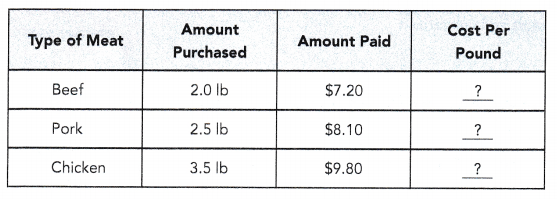Which type of meat costs the least per pound?
Chicken.
Explanation: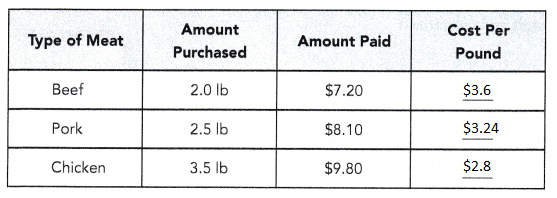From the above table Chicken costs the least amount per pound.

Question 11.
Math Journal The table shows the data about distances and times for three sprinters.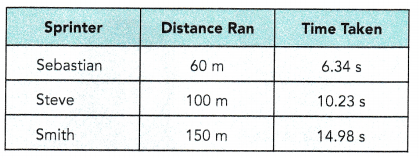Smith is the fastest sprinter
Explanation: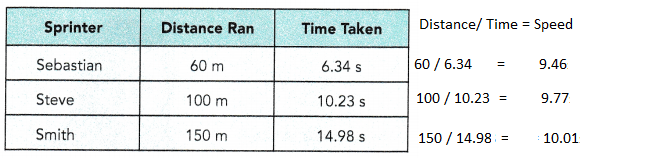Question 12.
A supermarket sells the three brands of rice shown in the table below.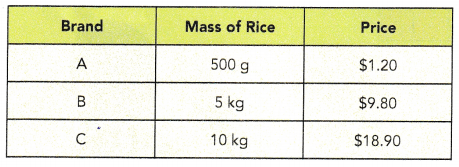Raimondo wants to buy 30 kilograms of rice.
a) Which brand of rice should he buy to get the best deal, assuming that all three brands are of the same quality?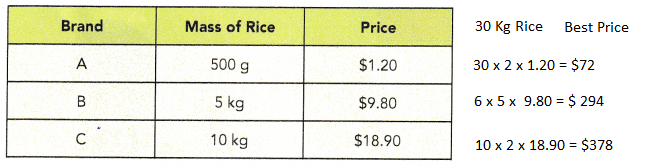$306 Explanation: Cheapest brand price =$72